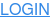# Python using logsense-logger

### Step 1: Install the plugin

``pip3 install logsense-logger``

### Step 2: Use it

#### Plain logging

An example of using the plugin for regular logging purposes is following:

``````from logsense.handler import LogSenseHandler
from os import getenv
import logging

logsense_token = getenv('LOGSENSE_TOKEN', None)
logging.getLogger().setLevel(logging.INFO)

class Example:
def __init__(self):
self.bar = 42

def foo(self):

if __name__ == "__main__":
ex = Example()
ex.foo()``````

Now, just run the app, e.g.

``LOGSENSE_TOKEN="aaaaaa-1111-bbbbbb" python sample_logging.py``

And your logs should flow to LogSense.

#### Metrics

The package also includes a simple example on measuring method duration. All that needs to be done is providing `LOGSENSE_TOKEN` environment variable and than annotating methods that are to be measure with `@measure_duration()`. For example:

``````from logsense.metrics import measure_duration, setup_metrics
from os import getenv

logsense_token = getenv('LOGSENSE_TOKEN')
setup_metrics('myapp', logsense_token)

class MyComplexProcess:
def __init__(self, how_many_times):
self._how_many_times = how_many_times

@measure_duration(extracted_params=['a'])
def foo(self, a):
x = 0
for i in range(a*self._how_many_times):
x = x+1
return x``````

Now, execution duration of `foo` will be tracked and sent to LogSense.

##### Example

To run it, execute following:

``````\$ pip install Flask
``\$ ./benchmark.py``